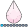## Plot of means

Hi,

I am doing project about how affect meals on blood glucose.

I have following code:

``````DATA glucose;
infile 'E:\GLUCOSE.DAT' EXPANDTABS;
input id g1-g10;
tm=int((_n_-1)/6)+1;
run;

DATA missqlucose;
SET glucose;
if g9=-1 then g9=.;
if g10=-1 then g10=.;
run;

PROC plot data=missqlucose (firstobs = 1 obs = 6);
run;

DATA glucose1;
set missqlucose (firstobs = 1 obs = 6);
run;

PROC TRANSPOSE data=glucose1 out=gtrans1 name=time prefix=p;
VAR g1-g10;
run;

PROC PRINT DATA= gtrans1;
run;``````

Now i need to make one plot of the separate tm class means across gk, where k is from 1:10 (in other words, for g1 g2 g3....g10)

P.S. i attached fili that in infile (see above), just change *.txt to *.dat

2 REPLIES 2djrisks
Barite | Level 11

## Re: Plot of means

Hello,

If you just want the mean then you can use the vbar statement in Proc SGPLOT. Below is the code that you can use to get this. I wasn't sure if you wanted means of g1-g10 or means of p1-p6, but you can adjust the code accordingly.

PS, it may be useful to see the variation in the data, so you could also look at doing a boxplot. or plotting the mean and confidence intervals.

proc sort data = gtrans1;
by time;
run;

proc transpose data = gtrans1 out = gtrans_stacked;
var p1 - p6;
by time;
run;

proc sgplot data = gtrans_stacked;
vbar _NAME_ / response = COL1 stat= mean;
run;

## Re: Plot of means

Hi,

Thank you for code. How to put values of means next to each bar on that graph?

Discussion stats
• 2 replies
• 920 views
• 2 likes
• 2 in conversation